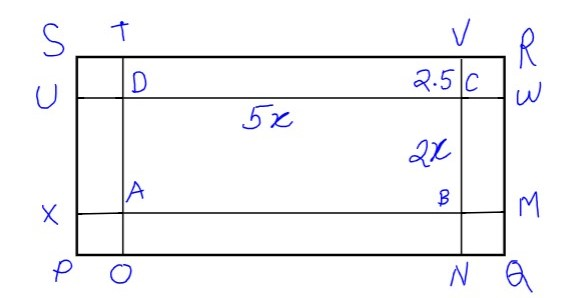QuestionAnswers

# The length and breadth of a rectangular park are in the ratio 5:2 a 2.5m path running all around the outside of the park has an area of $305{m^2}$ . Find the dimensions of the park.Hint: Try to get an equation in x, using the area of the path outside the park by dividing the path in 4 small rectangles and finding the area individually and then at last adding them all to get $305{m^2}$ . In this way we can get the value of x and then putting it in $5x$ & $2x$ we can get the dimensions of the park.According to the question it is given that there's a 2.5m path running all along the perimeter of the rectangular park
Therefore, $TD = DU = VC = WC = XA = AO = BN = BM = 2.5m$
Again from the Figure it is clear that $SR = UW = XM = PQ$ and also
$SP = TO = VN = RQ$
As $CB = AD = 2x$ & $DC = AB = 5x$
Then, $UW = XM = 5x + 5$
Again it can be observed that Area of rectangle UWRS = Area of rectangle XMQP
Also, Area of rectangle UDAX = Area of rectangle CWMB
$\therefore$ Area of Path = Area of UDAX + Area of CWMB + Area of XMQP + Area of UWRS
$\therefore$ Area of Path = 2(Area of UDAX + Area of XMQP)
Now Area of UDAX = $UD \times AX = 2x \times 2.5 = 5x$
Area of XMQP = $XM \times MQ = 2.5(5x + 5)$
$\therefore$ Area of Path = $305{m^2}$
$\begin{array}{l} \Rightarrow 305 = 2\{ 2.5(5x + 5) + 5x\} \\ \Rightarrow 305 = 5(5x + 5) + 10x\\ \Rightarrow 305 = 25x + 25 + 10x\\ \Rightarrow 305 = 35x + 25\\ \Rightarrow 280 = 35x\\ \Rightarrow x = 8 \end{array}$
Now as we have the value of $x = 8$ Then it is given that dimension are 5x and 2x
$\therefore 5x = 40$ & $2x = 10$
Therefore the dimensions of the rectangular park are 10m and 40m.

Note: One must be careful while observing the diagram because that is the key to the whole question and also while adding the length of the path in both sides of the rectangular sides. For the side CD(rectangular side) the lengths CW and DU must be added separately, a lot of students missed that and found the wrong answer. Also you can extend the sides of CB and AD as TO and VN which will be $2x + 5$ and then by proceeding in the same manner we can get the correct answer.

Relation Between the Length of a Given Wire and Tension for Constant Frequency Using SonometerWhat are the Functions of the Human Skeletal System?What are the Successor and Predecessor?Measurement of Length DistanceThe Making of a ScientistChanging the Period of a PendulumCBSE Class 9 Maths Chapter 6 - Lines and Angles FormulasCBSE Class 9 Maths Chapter 13 - Surface Areas and Volumes FormulasCBSE Class 9 Maths FormulasTable of 9 - Multiplication Table of 9Important Questions for CBSE Class 9 Maths Chapter 9 - Areas of Parallelograms and TrianglesImportant Questions for CBSE Class 7 English An Alien Hand Chapter 9 - A Tiger In The HouseImportant Questions for CBSE Class 6 English A Pact with The Sun Chapter 9 - What Happened to The ReptilesImportant Questions for CBSE Class 6 English A Pact with The Sun Chapter 6 - The Monkey and the CrocodileImportant Questions for CBSE Class 9 Science Chapter 4 - Structure of The AtomImportant Questions for CBSE Class 9 Science Chapter 9 - Force and Laws of MotionImportant Questions for CBSE Class 6 English A Pact with The Sun Chapter 8 - A Pact with the SunImportant Questions for CBSE Class 9 Science Chapter 5 - The Fundamental Unit of LifeImportant Questions for CBSE Class 6 English A Pact with The Sun Chapter 1 - A Tale of Two BirdsImportant Questions for CBSE Class 7 English Honeycomb Chapter 9 - A Bicycle In Good RepairCBSE Class 10 Hindi A Question Paper 2020Hindi A Class 10 CBSE Question Paper 2009Hindi A Class 10 CBSE Question Paper 2015Hindi A Class 10 CBSE Question Paper 2016Hindi A Class 10 CBSE Question Paper 2012Hindi A Class 10 CBSE Question Paper 2010Hindi A Class 10 CBSE Question Paper 2007Hindi A Class 10 CBSE Question Paper 2013Hindi A Class 10 CBSE Question Paper 2008Hindi A Class 10 CBSE Question Paper 2014RS Aggarwal Class 9 Solutions Chapter-9 Congruence of Triangles and Inequalities in a TriangleNCERT Solutions for Class 9 Maths Chapter 9 Areas of Parallelograms and TrianglesRD Sharma Solutions for Class 9 Maths Chapter 18 - Surface Area and Volume of a Cuboid and CubeRD Sharma Solutions for Class 9 Maths Chapter 21 - Surface Area and Volume of a SphereNCERT Solutions for Class 9 English Beehive Chapter 5 - A Legend of The NorthlandNCERT Solutions For Class 9 Science Chapter 9RD Sharma Solutions for Class 9 Maths Chapter 9 - Triangle and its AnglesRD Sharma Solutions for Class 9 Maths Chapter 19 - Surface Area and Volume of A Right Circular CylinderRD Sharma Solutions for Class 9 Maths Chapter 20 - Surface Area and Volume of A Right Circular ConeNCERT Solutions for Class 9 Maths Chapter 9 Area Of Parrallelograms and triangles In Hindi